# Introduction to Data Visualization in Python¶

Author: Sean Trott

The goal of this tutorial is to familiarize you with the basics of data visualization in Python.

Note that the slides version can be found here, and the repository itself can be found here.

## What is data visualization?¶

Data visualization refers to the process (and result) of representing data graphically.

For our purposes today, we'll be talking mostly about common methods of plotting data, including:

• Histograms
• Scatterplots
• Line plots
• Bar plots

## Why is data visualization important?¶

#### Exploratory data analysis¶

• Checking assumptions: does my data look like how I expect it to look?
• Generating hypotheses: what relationships can I discover in the data?

#### Communicating insights¶

• Communicating information clearly, concisely, and accurately.

#### Impacting the world¶

• Data visualizations can (and have) played a big role in shaping policy, business decisions, and more.

### Exploratory Data Analysis: Checking your assumptions¶

Anscombe's Quartet### Communicating Insights¶

Reference: Full Stack Economics## Impacting the world¶

Florence Nightingale (1820-1910) was a social reformer, statistician, and founder of modern nursing.## So how do we visualize data?¶

In today's tutorial, we'll discuss how to visualize data using Python.

## Key learning outcomes¶

• Describe the benefits and advantages of data visualization.
• Propose a suitable data visualization for a particular dataset and question.
• Implement a first-draft of this visualization in Python, using either matplotlib or seaborn.
• Evaluate this data visualization and make suggestions for improvements in future iterations.
• Reconstruct a visualization found "in the wild" using the appropriate dataset.

Here, we load the core packages we'll be using:

We also add some lines of code that make sure our visualizations will plot "inline" with our code, and that they'll have nice, crisp quality.

In :
import pandas as pd # conventionalized abbreviation
import matplotlib.pyplot as plt # conventionalized abbreviation
import seaborn as sns # conventionalized abbreviation

/Users/seantrott/anaconda3/lib/python3.7/site-packages/pandas/compat/_optional.py:138: UserWarning: Pandas requires version '2.7.0' or newer of 'numexpr' (version '2.6.8' currently installed).
warnings.warn(msg, UserWarning)

In :
%matplotlib inline
%config InlineBackend.figure_format = 'retina'


## Part 1: Basic plot types with matplotlib.pyplot¶

In this section, we'll explore several basic plot types, first using matplotlib.pyplot. These will include:

• Histograms
• Scatterplots
• Barplots

Afterwards (in Part 2), we will discuss how to replicate these plot types with seaborn, an API built on top of pyplot.

To get started, we'll load a dataset from seaborn.

In :
## Load taxis dataset
len(df_taxis)

Out:
6433
In :
## What are the columns/variables in this dataset?

Out:
pickup dropoff passengers distance fare tip tolls total color payment pickup_zone dropoff_zone pickup_borough dropoff_borough
0 2019-03-23 20:21:09 2019-03-23 20:27:24 1 1.60 7.0 2.15 0.0 12.95 yellow credit card Lenox Hill West UN/Turtle Bay South Manhattan Manhattan
1 2019-03-04 16:11:55 2019-03-04 16:19:00 1 0.79 5.0 0.00 0.0 9.30 yellow cash Upper West Side South Upper West Side South Manhattan Manhattan
2 2019-03-27 17:53:01 2019-03-27 18:00:25 1 1.37 7.5 2.36 0.0 14.16 yellow credit card Alphabet City West Village Manhattan Manhattan
3 2019-03-10 01:23:59 2019-03-10 01:49:51 1 7.70 27.0 6.15 0.0 36.95 yellow credit card Hudson Sq Yorkville West Manhattan Manhattan
4 2019-03-30 13:27:42 2019-03-30 13:37:14 3 2.16 9.0 1.10 0.0 13.40 yellow credit card Midtown East Yorkville West Manhattan Manhattan

### Histograms: plotting frequency distributions¶

Histograms are critical for visualizing how your data distribute.

They are typically used for continuous, quantitative variables. Examples could include: height, income, and temperature.

The pyplot package has a dedicated function for creating histograms, called hist (documentation here).

An example call would look like this:

plt.hist(
x = [ ... ],
bins = 10, # set number of bins
density = False, # indicate whether to draw density plot
alpha = .7 # indicate shading/transparency of plot
)

Now let's plot some of our variables from the taxis dataset!

In :
## Q: How would you describe the shape of this distribution?

plt.hist(
x=df_taxis['fare'], # this is the variable we're plotting
bins=20, # this is the number of bins we want to know
alpha = .5 # this is our alpha level
)
plt.show()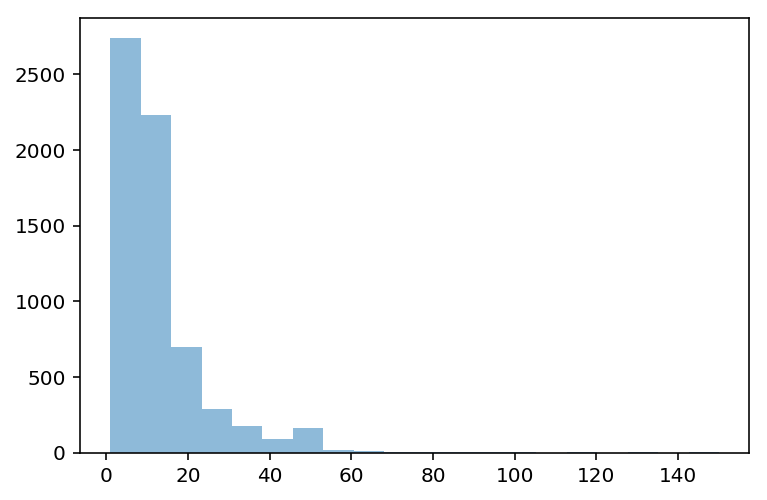We can also add axis labels, so it's clear what we're plotting.

In :
plt.hist(
x=df_taxis['fare'], # this is the variable we're plotting
bins=20, # this is the number of bins we want to know
alpha = .5 # this is our alpha level
)
plt.xlabel("Fare ($)") plt.ylabel("Count") plt.title("Distribution of Taxi Fares ($)")
plt.show()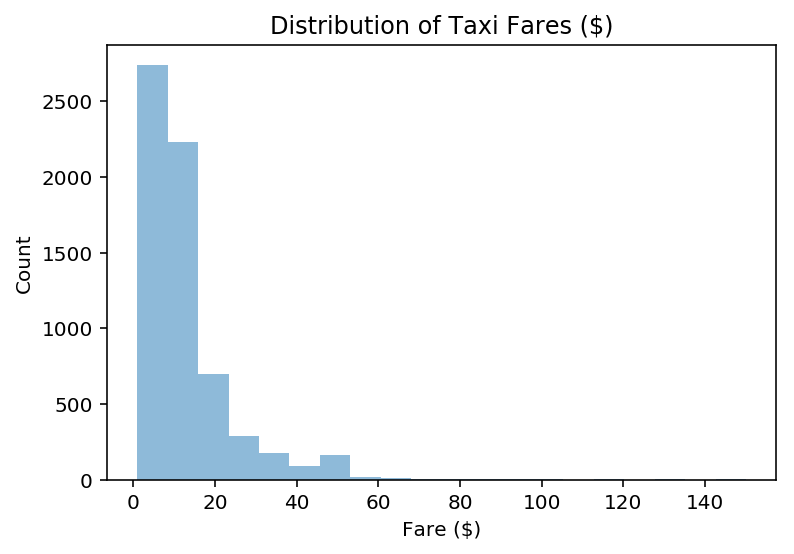#### Q: How would you plot the distribution of trip distances?¶

In :
plt.hist(
x=df_taxis['distance'], # this is the variable we're plotting
bins=20, # this is the number of bins we want to know
alpha = .5 # this is our alpha level
)
plt.xlabel("Distance (miles)")
plt.ylabel("Count")
plt.title("Distribution of Trip Distances")
plt.show()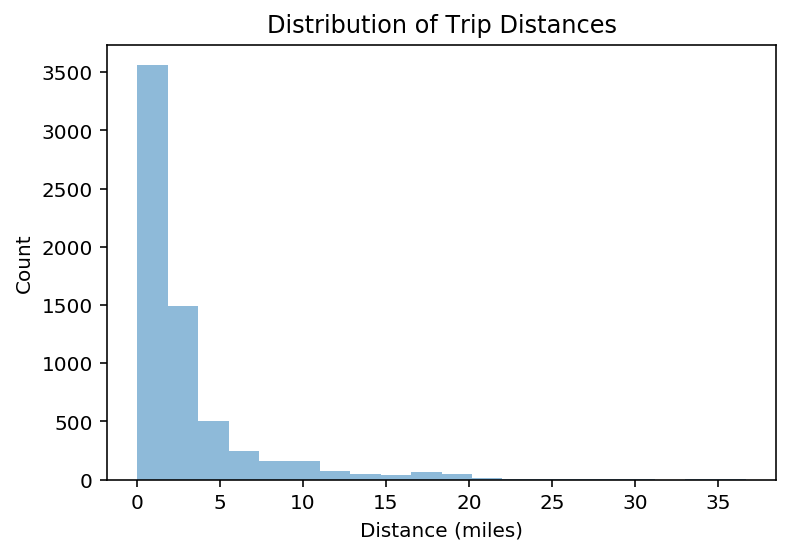#### Q: What about the distribution of tip amounts?¶

In :
plt.hist(
x=df_taxis['tip'], # this is the variable we're plotting
bins=20, # this is the number of bins we want to know
alpha = .5 # this is our alpha level
)
plt.xlabel("Tip ($)") plt.ylabel("Count") plt.title("Distribution of Tip Amounts ($)")
plt.show()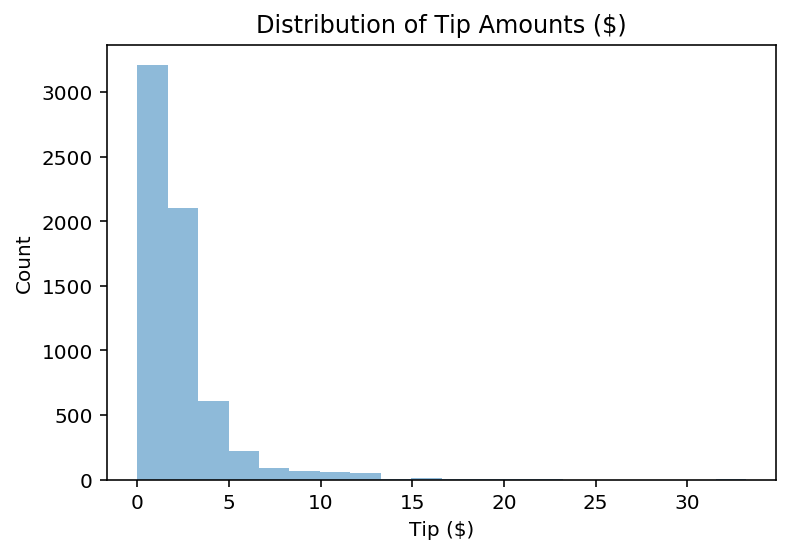#### Creating a new variable¶

Presumably, these tip amounts correlate with the overall fare. We might also want to know about the amount in percentage-terms, e.g., how much a customer tipped as a percentage of how much the fare was.

In :
## tip_pct = tip / fare
df_taxis['tip_pct'] = (df_taxis['tip'] / df_taxis['fare']) * 100


Now we can plot this new variable, to get a sense of the distribution of tip percentages.

Q: What conclusions might we draw about the distribution of tip percentages here?

In :
plt.hist(
x=df_taxis['tip_pct'], # this is the variable we're plotting
bins=20, # this is the number of bins we want to know
alpha = .5 # this is our alpha level
)
plt.xlabel("Tip (%)")
plt.ylabel("Count")
plt.title("Distribution of Tip Percentages (%)")
plt.show()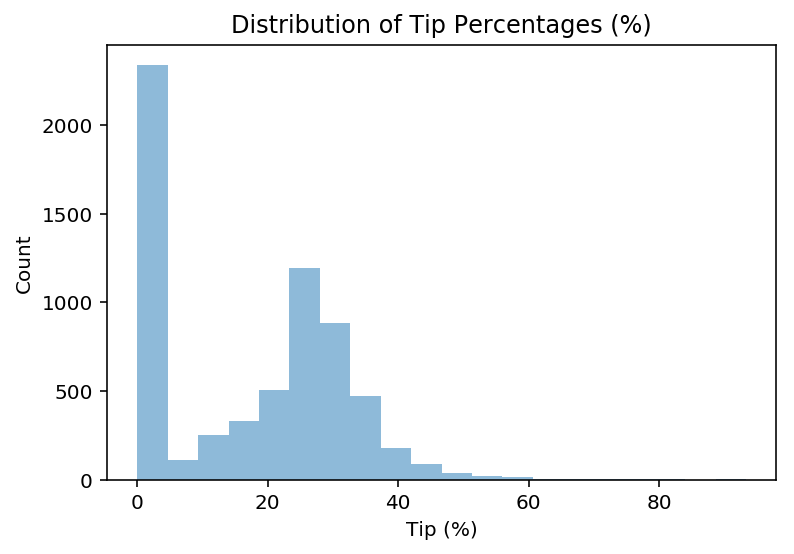#### Q: Adding other markers to the plot?¶

What if we wanted to add more information to this plot, such as the mean tip percentage?

(Hint: use either plt.axvline or plt.text).

In :
# First, calculate mean tip for ease of access
MEAN_TIP = df_taxis['tip_pct'].mean()

In :
# Drawing a vertical line

plt.hist(
x=df_taxis['tip_pct'], # this is the variable we're plotting
bins=20, # this is the number of bins we want to know
alpha = .5 # this is our alpha level
)

plt.axvline(MEAN_TIP, linestyle = "dotted")

plt.xlabel("Tip (%)")
plt.ylabel("Count")
plt.title("Distribution of Tip Percentages (%)")
plt.show()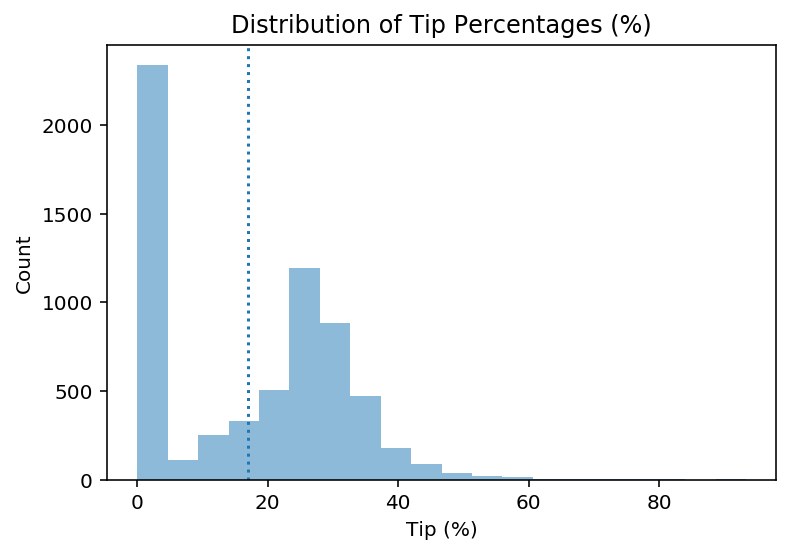In :
# Annotating with text

plt.hist(
x=df_taxis['tip_pct'], # this is the variable we're plotting
bins=20, # this is the number of bins we want to know
alpha = .5 # this is our alpha level
)

plt.text(MEAN_TIP, # where on x-axis to display
y = 1500, # where on y-axis to display
s = "Mean Tip: {mean}%".format(mean=str(round(MEAN_TIP, 2))))

plt.xlabel("Tip (%)")
plt.ylabel("Count")
plt.title("Distribution of Tip Percentages (%)")
plt.show()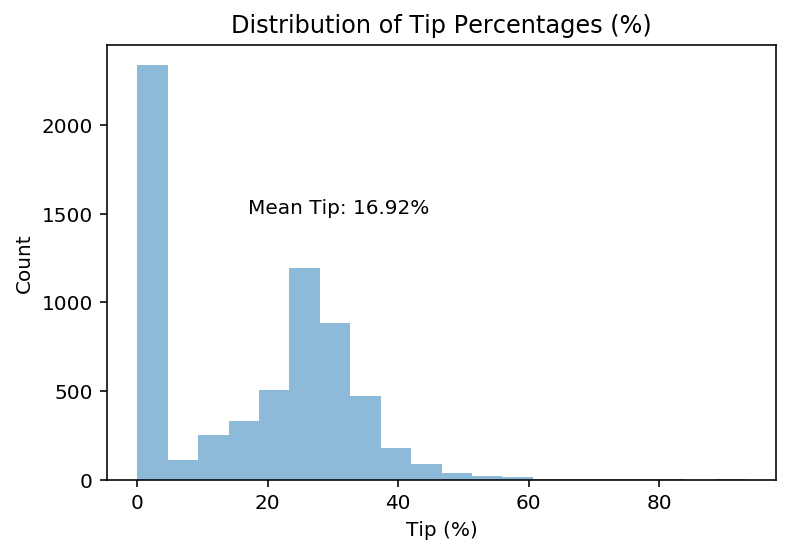### Scatterplots: plotting multiple continuous variables¶

Scatterplots can be used to look at how two different continuous variables relate to each other.

Among other things, scatterplots are critical for exploratory data analysis––for example, if you want to run a linear regression on your dataset, it is important to determine that the relationship you're investigating is linear (remember Anscombe's Quartet).

The pyplot package has a dedicated function for creating scatterplots as well, called scatter (documentation here).

An example call would look like this:

plt.scatter(
x = [ ... ], # array of values for x-axis
y = [ ... ], # array of values for y-axis
c = [ ... ], # array of values to indicate color of points
alpha = .7 # indicate shading/transparency of plot
)

#### What to ask our data?¶

Intuitively, we might want to know a few things:

1. Do longer distances cost more?
2. Do people tip more (either in amount or percentage terms) for longer distances?
3. Does it cost more to travel more between boroughs, even holding the distance constant?
In :
# Q: Do longer distances cost more?

plt.scatter(
x = df_taxis['distance'],
y = df_taxis['fare'],
alpha = .6
)
plt.xlabel("Distance (miles)")
plt.ylabel("Fare ($)") plt.title("Raw Fare by Distance Traveled") plt.show()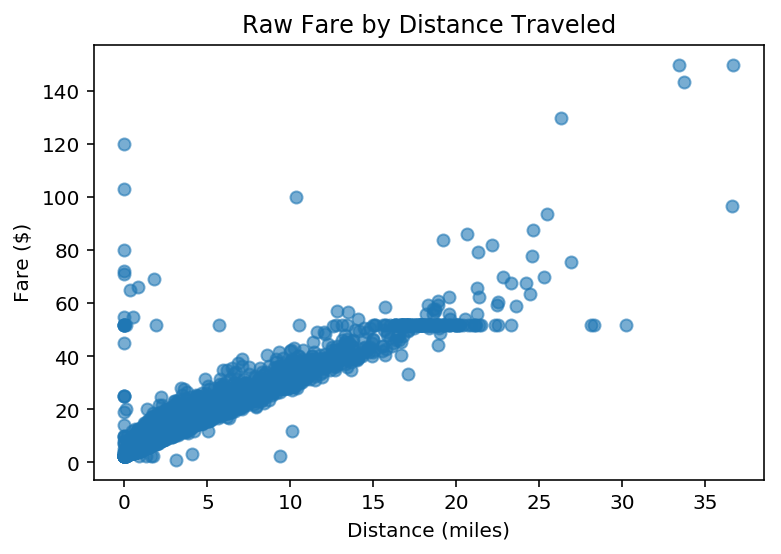#### Q: How could we ask about the relationship between distance and tip amount?¶ In : plt.scatter( x = df_taxis['distance'], y = df_taxis['tip'], alpha = .6 ) plt.xlabel("Distance (miles)") plt.ylabel("Tip ($)")
plt.title("Tip Amount by Distance Traveled")
plt.show()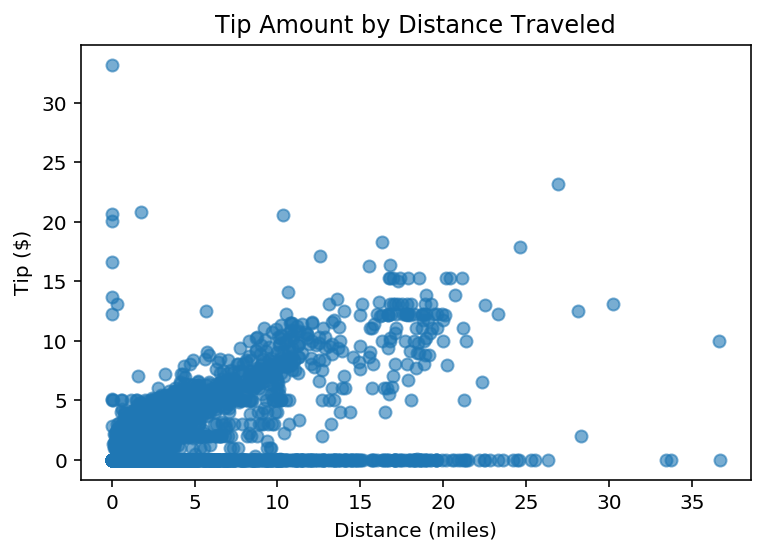#### Q: What about distance and tip percentage?¶

In :
plt.scatter(
x = df_taxis['distance'],
y = df_taxis['tip_pct'],
alpha = .4
)
plt.xlabel("Distance (miles)")
plt.ylabel("Tip (%)")
plt.title("Tip Percentage by Distance Traveled")
plt.show()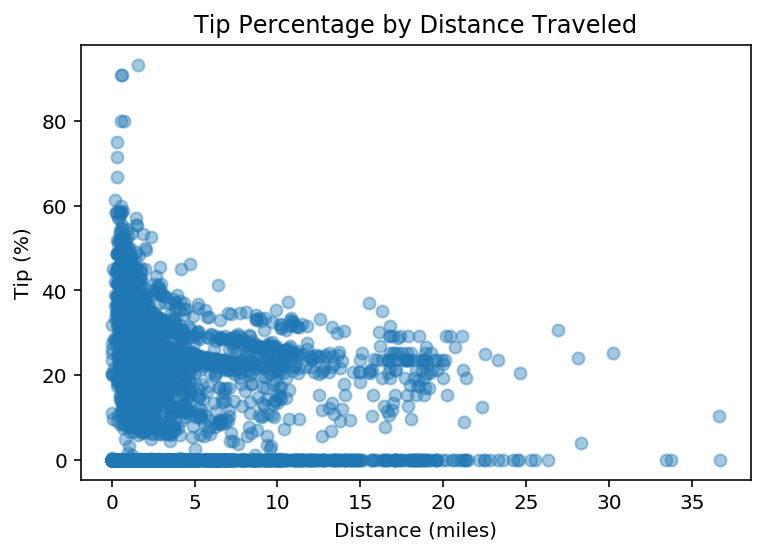#### Q: What about crossing between boroughs?¶

In :
## Create a new variable
df_taxis['crossed_borough'] = df_taxis['pickup_borough'] != df_taxis['dropoff_borough']

Out:
pickup dropoff passengers distance fare tip tolls total color payment pickup_zone dropoff_zone pickup_borough dropoff_borough tip_pct crossed_borough
0 2019-03-23 20:21:09 2019-03-23 20:27:24 1 1.6 7.0 2.15 0.0 12.95 yellow credit card Lenox Hill West UN/Turtle Bay South Manhattan Manhattan 30.714286 False

#### Plotting categorical and continuous data¶

Now, we want to add more information to our scatterplot––we have a categorical variable (crossed_borough), and we want the color (or perhaps shape) of each point to indicate the level of that variable, i.e., whether the trip crossed between boroughs.

In :
cdict = {True: 'orange', False: 'blue'}
fig, ax = plt.subplots()
for group in set(df_taxis['crossed_borough']):
df_tmp = df_taxis[df_taxis['crossed_borough']==group] # filter to appropriate group
ax.scatter(
x = df_tmp['distance'],
y = df_tmp['fare'],
label = group,
c = cdict[group],
alpha = .3
)
plt.xlabel("Distance")
plt.ylabel("Fare (\$)")
ax.legend(title = "Crossed Borough")
plt.show()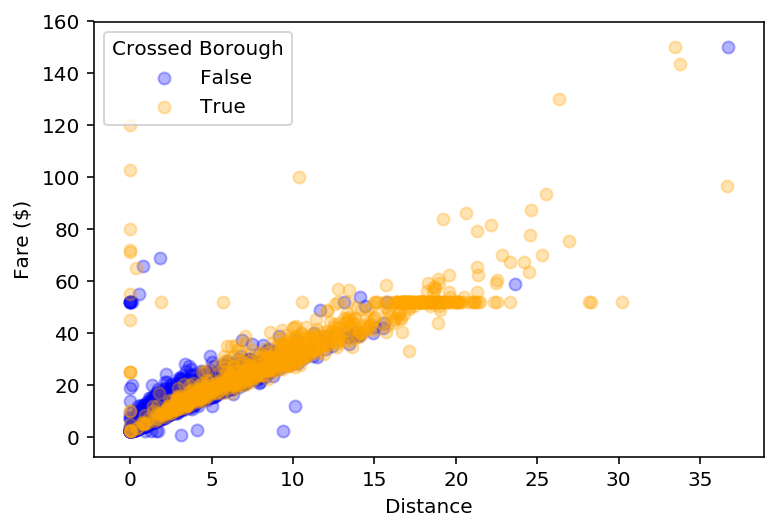In :
cdict = {True: 'orange', False: 'blue'}
fig, ax = plt.subplots()
for group in set(df_taxis['crossed_borough']):
df_tmp = df_taxis[df_taxis['crossed_borough']==group] # filter to appropriate group
ax.scatter(
x = df_tmp['distance'],
y = df_tmp['tip_pct'],
label = group,
c = cdict[group],
alpha = .3
)
plt.xlabel("Distance")
plt.ylabel("Tip (%)")
ax.legend(title = "Crossed Borough")
plt.show()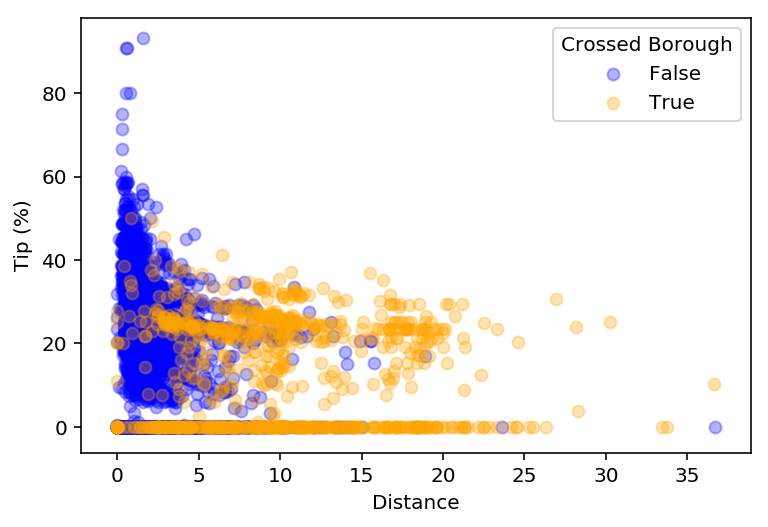### Barplots¶

Another commonly found type of plot is a barplot.

Typically, barplots feature:

• On the x-axis, a categorical (i.e., nominal) variable.
• On the y-axis, a continuous variable, usually summarized in some way (e.g., the means, with standard errors).

Barplots are effective for drawing attention to differences in the magnitude of some quantity across categories––though personally, I don't think they're always the most effective in terms of the amount of information conveyed per unit of space.

### Barplots in pyplot¶

The corresponding pyplot function is bar. We saw this earlier, when we were trying to plot the counts of a categorical variable.

The basic inputs are as follows:

plt.bar(
x = [ ... ], # array of categories
height = [ ... ] # height of each category
)

#### Creating a new dataframe using groupby¶

Once again, groupby is very helpful here. We'll want to:

1. Calculate the mean tip_pct for each level of the color variable.
2. Plot this using plt.bar.
In :
df_color_tips_mean = df_taxis[['tip_pct', 'color']].groupby('color').mean().reset_index()
df_color_tips_mean

Out:
color tip_pct
0 green 7.441110
1 yellow 18.627966
In :
# Q: What's missing from this graph?

plt.bar(x = df_color_tips_mean['color'],
height = df_color_tips_mean['tip_pct'])
plt.xlabel("Taxi Color")
plt.ylabel("Tip (%)")
plt.title("Tip (%) by Taxi Color")

Out:
Text(0.5, 1.0, 'Tip (%) by Taxi Color')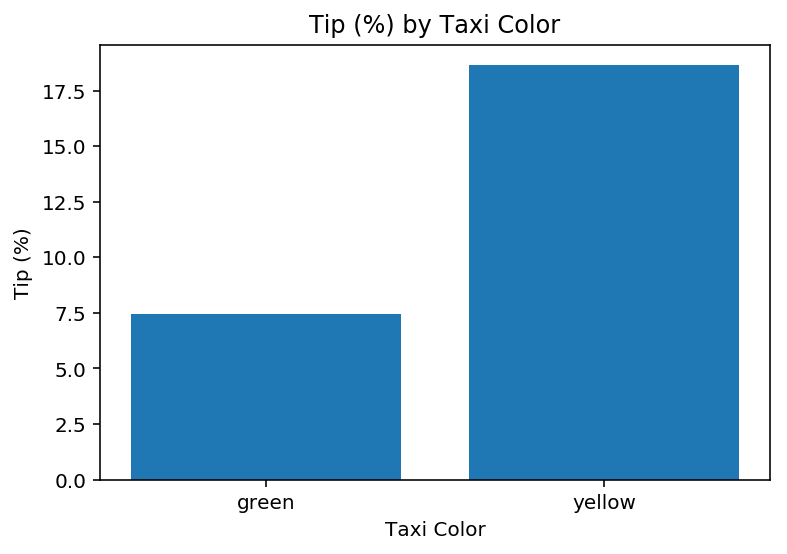#### Adding error bars¶

To add error bars, we can use plt.errorbar.

In :
## First, calculate standard error of the mean
df_color_tips_sem = df_taxis[['tip_pct', 'color']].groupby('color').sem().reset_index()
df_color_tips_sem

Out:
color tip_pct
0 green 0.384112
1 yellow 0.194550
In :
plt.bar(x = df_color_tips_mean['color'],
height = df_color_tips_mean['tip_pct'])

plt.errorbar(x = df_color_tips_mean['color'], ## as with bar, the x-axis is the *color*
y = df_color_tips_mean['tip_pct'], ## as with bar, the y-axis is the *mean*
yerr = df_color_tips_sem['tip_pct'] * 2, ## two standard errors, as typical
ls = 'none', ## toggle this to connect or not connect the lines
color = "black")

plt.xlabel("Taxi Color")
plt.ylabel("Tip (%)")
plt.title("Tip (%) by Taxi Color")

Out:
Text(0.5, 1.0, 'Tip (%) by Taxi Color')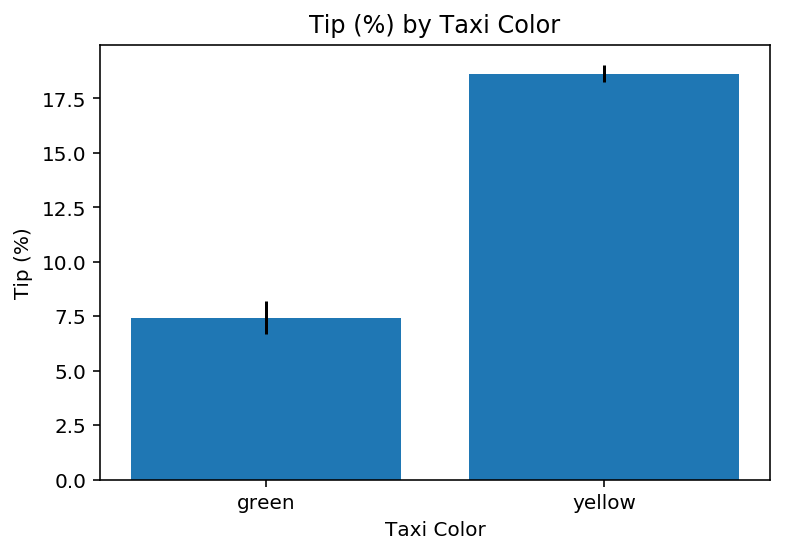### Other pyplot affordances¶

This short tutorial really only scratched the surface of what you can do with pyplot.

Just to give a sense:

## Part 2: Plotting with seaborn¶

In this section, we'll replicate some of those same plots from above, but now using seaborn.

As you'll see, seaborn offers an API that's (in most cases) cleaner and easier to use than pyplot, though there's a trade-off in terms of flexibility.

### Histograms¶

Depending on your version of seaborn, you can plot a histogram using either:

• seaborn.distplot: typically used for creating density plots, but can be adapted for histograms.
• seaborn.histplot: targeted more specifically at histograms.
In :
sns.distplot(df_taxis['distance'], ## vector to plot
kde = False, ## whether to use kernel density estimation to fit density curve
norm_hist = False ## whether to norm histogram to display probability vs. counts
)
plt.xlabel("Distance (miles)")
plt.ylabel("Count")
plt.title("Distribution of miles traveled")

Out:
Text(0.5, 1.0, 'Distribution of miles traveled')